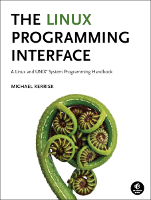This is timers/ptmr_sigev_thread.c (Listing 23-7, page 506), an example from the book, The Linux Programming Interface.

This page shows the "distribution" or "book" version of the file (why are there two versions?), or the differences between the two versions. You can switch between the views using the tabs below.

In the listing below, the names of Linux system calls and C library functions are hyperlinked to manual pages from the Linux man-pages project, and the names of functions implemented in the book are hyperlinked to the implementations of those functions.Function list (Bold in this list means a function is not static)

for expirations of a POSIX timer. Each of the program's command-line
arguments specifies the initial value and interval for a POSIX timer. The
format of these arguments is defined by the function itimerspecFromStr().

The program creates and arms one timer for each command-line argument.
as its start function. The threadFunc() function displays information
about the timer expiration, increments a global counter of timer expirations,
and signals a condition variable to indicate that the counter has changed.
In the main thread, a loop waits on the condition variable, and each time
the condition variable is signaled, the main thread prints the value of the
global variable that counts timer expirations.

Kernel support for Linux timers is provided since Linux 2.6. On older
systems, an incomplete user-space implementation of POSIX timers
was provided in glibc.
*/
#include <signal.h>
#include <time.h>
#include "curr_time.h"              /* Declares currTime() */
#include "tlpi_hdr.h"
#include "itimerspec_from_str.h"    /* Declares itimerspecFromStr() */

static int expireCnt = 0;           /* Number of expirations of all timers */
{
timer_t *tidptr;
int s;

tidptr = sv.sival_ptr;

printf("    timer ID=%ld\n", (long) *tidptr);
printf("    timer_getoverrun()=%d\n", timer_getoverrun(*tidptr));

/* Increment counter variable shared with main thread and signal
condition variable to notify main thread of the change. */

if (s != 0)

expireCnt += 1 + timer_getoverrun(*tidptr);

if (s != 0)

if (s != 0)
}
int
main(int argc, char *argv[])
{
struct sigevent sev;
struct itimerspec ts;
timer_t *tidlist;
int s, j;

if (argc < 2)
usageErr("%s secs[/nsecs][:int-secs[/int-nsecs]]...\n", argv);

tidlist = calloc(argc - 1, sizeof(timer_t));
if (tidlist == NULL)
errExit("malloc");

sev.sigev_notify_attributes = NULL;
/* Could be pointer to pthread_attr_t structure */

/* Create and start one timer for each command-line argument */

for (j = 0; j < argc - 1; j++) {
itimerspecFromStr(argv[j + 1], &ts);

sev.sigev_value.sival_ptr = &tidlist[j];
/* Passed as argument to threadFunc() */

if (timer_create(CLOCK_REALTIME, &sev, &tidlist[j]) == -1)
errExit("timer_create");
printf("Timer ID: %ld (%s)\n", (long) tidlist[j], argv[j + 1]);

if (timer_settime(tidlist[j], 0, &ts, NULL) == -1)
errExit("timer_settime");
}

/* The main thread waits on a condition variable that is signaled
print a message so that the user can see that this occurred. */

if (s != 0)